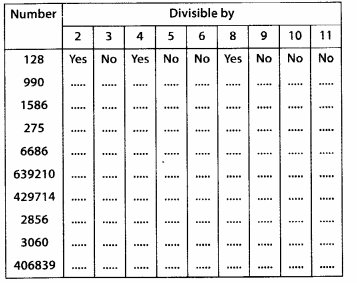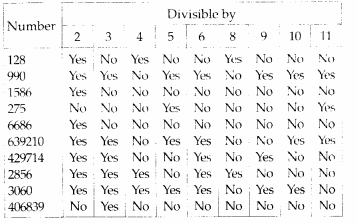# MP Board Class 6th Maths Solutions Chapter 3 Playing With Numbers Ex 3.3

## MP Board Class 6th Maths Solutions Chapter 3 Playing With Numbers Ex 3.3

Question 1.
Using divisibility tests, determine which of the following numbers are divisible by 2; by 3; by 4; by 5; by 6; by 8; by 9; by 10; by 11 (say, yes or no):Solution:Question 2.
Using divisibility tests, determine which of the following numbers are divisible by 4; by 8:
(a) 572
(b) 726352
(c) 5500
(d) 6000
(e) 12159
(f) 14560
(g) 21084
(h) 31795072
(i) 1700
(j) 2150
Solution:
(a) 572 is divisible by 4 as its last two digits are divisible by 4, but it is not divisible
by 8 as its last three digits are not divisible by 8.
(b) 726352 is divisible by 4 as its last two digits are divisible by 4 and it is also divisible by 8 as its last three digits are divisible by 8.
(c) 5500 is divisible by 4 as its last two digits are divisible by 4, but it is not divisible by 8 as its last three digits are not divisible by 8.
(d) 6000 is divisible by 4 as its last two digits are divisible by 4 and it is also divisible by 8 as its last three digits are divisible by 8.
(e) 12159 is not divisible by 4 and 8 as it is an odd number.
(f) 14560 is divisible by 4 as its last two digits are divisible by 4 and it is also divisible by 8 as its last three digits are divisible by 8.
(g) 21084 is divisible by 4 as its last two digits are divisible by 4, but it is not divisible by 8 as its last three digits are not divisible by 8.
(h) 31795072 is divisible by 4 as its last two digits are divisible by 4 and it is also divisible by 8 as its last three digits are divisible by 8.
(i) 1700 is divisible by 4 as its last two digits are divisible by 4, but it is not divisible by 8 as its last three digits are not divisible by 8.
(j) 2150 is not divisible by 4 as its last two digits are not divisible by 4 and it is not divisible by 8 as its last three digits are not divisible by 8.Question 3.
Using divisibility tests, determine which of following numbers are divisible by 6:
(a) 297144
(c) 4335
(e) 901352
(f) 438750
(g) 1790184
(h) 12583
(i) 639210
(j) 17852
Solution:
(a) 297144 is divisible by 2 as its ones place is an even number place is an even number and it is also divisible by 3 as sum of its digits (= 27) is divisible by 3.
Since, the number is divisible by both 2 and 3. Therefore, it is also divisible by 6.

(b) 1258 is divisible by 2 as its ones place is an even number, but it is not divisible by 3 as sum of its digits (= 16) is not divisible by 3.
Since, the number is not divisible by both 2 and 3. Therefore, it is not divisible by 6.

(c) 4335 is not divisible by 2 as its ones place is not an even number, but it is divisible by 3 as sum of its digits (= 15) is divisible by 3.
Since, the number is not divisible by both 2 and 3. Therefore, it is not divisible by 6.

(d) 61233 is not divisible by 2 as its ones place is not an even number, but it is divisible by 3 as sum of its digits (= 15) is divisible by 3.
Since, the number is not divisible by both 2 and 3. Therefore, it is not divisible by 6.

(e) 901352 is divisible by 2 as its ones place is an even number, but it is not divisible by 3 as sum of its digits (= 20) is not divisible by 3.
Since, the number is not divisible by both 2 and 3. Therefore, it is not divisible by 6.

(f) 438750 is divisible by 2 as its ones place
is an even number and it is also divisible by 3 as sum of its digits (= 27) is divisible by 3.
Since, the number is divisible by both 2 and 3. Therefore, it is also divisible by 6.

(g) 1790184 is divisible by 2 as its ones place is an even number and it is also divisible by 3 as sum of its digits (= 30) is divisible by 3.
Since, the number is divisible by both 2 and 3. Therefore, it is also divisible by 6.

(h) 12583 is not divisible by 2 as its ones place is not an even number and it is also not divisible by 3 as sum of its digits (= 19) is not divisible by 3.
Since, the number is not divisible by both 2 and 3. Therefore, it is not divisible by 6.

(i) 639210 is divisible by 2 as its ones place is an even number and it is also divisible by 3 as sum of its digits (= 21) is divisible by 3.
Since, the number is divisible by both 2 and 3. Therefore, it is divisible by 6.

(j) 17852 is divisible by 2 as its ones place is an even number, but it is not divisible by 3 as sum of its digits (= 23) is not divisible by 3.
Since, the number is not divisible by both 2 and 3. Therefore, it is not divisible by 6.Question 4.
Using divisibility tests, determine which of the following numbers are divisible by 11:
(a) 5445
(b) 10824
(c) 7138965
(d) 70169308
(e) 10000001
(f) 901153
Solution:
(a) In 5445, sum of the digits at odd places = 5 + 4 = 9
Sum of the digits at even places = 4 + 5 = 9
Difference of both sums = 9 – 9 = 0
Since the difference is 0. Therefore, the number is divisible by 11.

(b) In 10824, sum of the digits at odd places = 4 + 8 + 1 = 13
Sum of the digits at even places = 2 + 0 = 2
Difference of both sums = 13 – 2 = 11
Since the difference is divisible by 11.
Therefore, the number is divisible by 11.

(c) In 7138965, sum of the digits at odd places = 5 + 9 + 3 + 7 = 24
Sum of the digits at even places = 6 + 8 + 1 = 15
Difference of both sums = 24 – 15 = 9
Since the difference is neither 0 nor divisible by 11. Therefore, the number is not divisible by 11.

(d) In 70169308, sum of the digits at odd places = 8 + 3 + 6 + 0 = 17
Sum of tire digits at even places = 0 + 9 + 1 + 7 = 17
Difference of both sums = 17 – 17 = 0
Since the difference is 0. Therefore, the number is divisible by 11.

(e) In 10000001, sum of the digits at odd places = 1 + 0 + 0 + 0 = 1
Sum of the digits at even places =0 + 0 + 0 + 1 = 1
Difference of both sums = 1 – 1 = 0
Since the difference is 0. Therefore, the number is divisible by 11.

(f) In 901153, sum of the digits at odd places =3 + 1 + 0 = 4
Sum of the digits at even places = 5 + 1 + 9 = 15
Difference of both sums = 15 – 4 = 11
Since the difference is 11. Therefore, the number is divisible by 11.

Question 5.
Write the smallest digit and the greatest digit in the blank space of each of the following numbers so that the number formed is divisible by 3:
(a) _6724
(b) 4765 _2
Solution:
We know that a number is divisible by 3 if the sum of all digits is divisible by 3.
(a) The smallest digit will be 2.
∴ The number formed is 26724 and
2 + 6 + 7 + 2 + 4 = 21, which is divisible by 3.
And the greatest digit will be 8.
∴ The number formed is 86724 and
8 + 6 + 7 + 2 + 4 = 27, which is divisible by 3.

(b) The smallest digit will be 0.
∴ The number formed is 476502 and
4 + 7 + 6 + 5 + 0 + 2 = 24, which is divisible by 3.
And the greatest digit will be 9.
∴ The number formed is 476592 and
4 + 7 + 6 + 5 + 9 + 2 = 33, which is divisible by 3.Question 6.
Write a digit in the blank space of each of the following numbers so that the number formed is divisible by 11 :
(a) 92 _ 389
(b) 8 _ 9484
Solution:
(a) We know that a number is divisible by 11 if the difference of the sum of the digits at odd places and that of even places is either 0 or divisible by 11.
The number formed is 928389
Sum of digits at odd places = 9 + 3 + 2 = 14
Sum of digits at even places = 8 + 8 + 9 = 25
Their difference = 25 – 14 = 11, which is divisible by 11.

(b) We know that a number is divisible by 11 if the difference of the sum of the digits at odd places and that of even places is either 0 or divisible by 11.
The number formed is 869484 Sum of digits at odd places = 4 + 4 + 6 = 14
Sum of digits at even places = 8 + 9 + 8 = 25
Their difference = 25 – 14 = 11, which is divisible by 11.